## A circular placemat has 44 inches of braid around the edge. What is the area of the placemat to the nearest square inch? Use 3.14 for π.

Question

A circular placemat has 44 inches of braid around the edge. What is the area of the placemat to the nearest square inch? Use 3.14 for π.

Enter the correct answer in the box.

[ ]

in progress 0
5 months 2021-09-05T11:56:31+00:00 2 Answers 0 views 0

a ~ 154

a =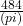Step-by-step explanation:

1. Approach

In this problem, one is given the circumference, the distance around the circle, one must find the area. In order to find the area, one must know the radius of the circle. To find the radius, one has to backsolve from the given circumference, then substitute the derived value into the formula for the area and solve.

The formula to find the circumference of a circle is;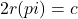Where (r) is the radius, (pi) is (3.1415), and (c) is the circumference.

Substitute in the given values and solve;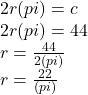3. Find the area

Now, one has the value of the radius, substitute it into the formula for the area of a circle and solve;

The formula for finding the area of a circle;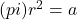Where (pi) is (3.1415), (r) is the radius, and (a) is the area,

Substitute in the value and solve.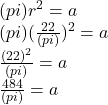a ~ 154

2. ### Answer:  approximately 154 square inches

==========================================================

Explanation:

We’ll need to use the circumference formula

C = 2*pi*r

to find the value of the radius r when C = 44

So we get

C = 2*pi*r

44 = 2*3.14*r

44 = 6.28*r

6.28r = 44

r = 44/6.28

r = 7.0063694267516

which is approximate

Then we use this value of r to find the area we need

A = pi*r^2

A = 3.14*(7.0063694267516)^2

A = 154.140127388536

A = 154

The area of the circular placemat is approximately 154 square inches.